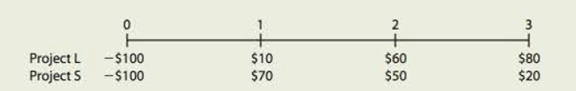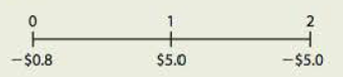Chapter 11, Problem 24ICFundamentals of Financial Manageme...

14th Edition
Eugene F. Brigham + 1 other
ISBN: 9781285867977

Solutions

Chapter
SectionFundamentals of Financial Manageme...

14th Edition
Eugene F. Brigham + 1 other
ISBN: 9781285867977
Textbook Problem

BASICS OF CAPITAL BUDGETING You recently went to work for Allied Components Company, a supplier of auto repair parts used in the after-market with products from Daimler AG, Ford, Toyota, and other automakers. Your boss, the chief financial officer (CFO), has just handed you the estimated cash flows for two proposed projects. Project L involves adding a new item to the firm’s ignition system line; it would take some time to build up the market for this product, so the cash inflows would increase over time. Project S involves an add-on to an existing line, and its cash flows would decrease over time. Both projects have 3-year lives because Allied is planning to introduce entirely new models after 3 years.Here are the projects’ after-tax cash flows (in thousands of dollars):Depredation, salvage values, net operating working capital requirements, and tax effects are all included in these cash flows. The CFO also made subjective risk assessments of each project, and he concluded that both projects have risk characteristics that are similar to the firm’s average project Allied’s WACC is 10%. You must determine whether one or both of the projects should be accepted. a. What is capital budgeting? Are there any similarities between a firm’s capital budgeting decisions and an individual’s investment decisions? b. What is the difference between independent and mutually exclusive projects? Between projects with normal and nonnormal cash flows? c. 1. Define the term net present value (NPV). What is each project’s NPV? 2. What is the rationale behind the NPV method? According to NPV, which project(s) should be accepted if they are independent? Mutually exclusive? 3. Would the NPVs change if the WACC changed? Explain. d. 1. Define the term internal rate of return (IRR). What is each project’s IRR? 2. How is the IRR on a project related to the YTM on a bond? 3. What is the logic behind the IRR method? According to IRR, which project(s) should be accepted if they are independent? Mutually exclusive? 4. Would the projects’ IRRs change if the WACC changed? e. 1. Draw NPV profiles for Projects L and S. At what discount rate do the profiles cross? 2. Look at your NPV profile graph without referring to the actual NPVs and IRRs. Which project(s) should be accepted if they are independent? Mutually exclusive? Explain. Are your answers correct at any WACC less than 23.6%? f. 1. What is the underlying cause of ranking conflicts between NPV and IRR? 2. What is the reinvestment rate assumption, and how does it affect the NPV versus IRR conflict? 3. Which method is best? Why? g. 1. Define the term modified IRR (MIRR). Find the MIRKs for Projects L and S. 2. What are the MIRR’s advantages and disadvantages as compared to the NPV? h. 1. What is the payback period? Find the paybacks for Projects L and S. 2. What is the rationale for the payback method? According to the payback criterion, which project(s) should be accepted if the firm’s maximum acceptable payback is 2 years, if Projects L and S are independent? If Projects L and S are mutually exclusive? 3. What is the difference between the regular and discounted payback methods? 4. What are the two main disadvantages of discounted payback? Is the payback method useful in capital budgeting decisions? Explain. i. As a separate project (Project P), the firm is considering sponsoring a pavilion at the upcoming World’s Fair. The pavilion would cost $800,000, and it is expected to result in$5 million of incremental cash inflows during its 1 year of operation. However, it would then take another year, and \$5 million of costs, to demolish the site and return it to its original condition. Thus, Project P’s expected cash flows (in millions of dollars) look like this:The project is estimated to be of average risk, so its WACC is 10%. 1. What is Project P’s NPV? What is its IRR? Its MIRR? 2. Draw Project P’s NPV profile. Does Project P have normal or nonnormal cash flows? Should this project be accepted? Explain.

a.

Summary Introduction

To explain: The concept of capital budgeting and whether there is any similarity between a firm’s capital budgeting decisions or individual investment decisions.

Capital Budgeting:

It refers to the long-term investment decisions that have been taken by the top management of a company and that are irreversible in nature. These decisions require an investment of a large amount of cash of the company.

Explanation
• Capital budgeting is the long-term investment decisions which includes a great amount of investment and that are irreversible in nature,unlike an individual’s investment decision...

b.

Summary Introduction

To explain: The difference between mutually exclusive and independent projects and between normal and nonnormal cash flow projects.

c.

1.

Summary Introduction

To explain: The concept of NPV and calculation of NPV of each project.

Net Present Value (NPV):

It is a method under capital budgeting which includes the computation of the net present value of the project in which company is investing. The calculation is done by calculating the difference between the value of cash inflow and value of cash outflow after taking into consideration the discounted rate.

2.

Summary Introduction

To explain: The rationale behind NPV method and whether project L or S should be selected if they independent and when they are mutually exclusive.

3.

Summary Introduction

To explain: Whether the NPV changes with the change in WACC.

d.

1.

Summary Introduction

To explain: The concept of IRR and calculation of IRR of each project.

Internal Rate of Return (IRR):

It refers to the rate of return that is computed by the company to make a decision of selection of a project for investment. This rate provides the basis for selection of projects with a lower cost of capital and rejection of project with a higher cost of capital.

2.

Summary Introduction

To explain: The relationship of IRR on a project to the YTM on a bond.

3.

Summary Introduction

To explain: The logic behind the IRR method and whether the project L or S should be selected when they are an independent project and when they are mutually exclusive projects.

4.

Summary Introduction

To explain: Whether the project IRR change with the change in WACC.

e.

1.

Summary Introduction

To construct: NPV profile for both the projects and crossover rate.

Crossover Rate:

It refers to that discounted rate at which the NPV of the two projects becomes equal. It is a cost of capital of the project.

2.

Summary Introduction

To explain: Whether to accept project A or project B on the basis of NPV profile when they are independent or when they are mutually exclusive.

f.

1.

Summary Introduction

To explain: The underlying cause of ranking conflicts between IRR and NPV.

2.

Summary Introduction

To explain: the reinvestment rate assumption and the way it affects the NPV versus IRR conflicts.

3.

Summary Introduction

To explain: Whether the NPV or IR is a better method.

g.

1.

Summary Introduction

To explain: The concept of MIRR and calculation of MIRR for given projects.

Modified Internal Rate of Return (MIRR):

It refers to the rate of return that is computed by the company to make a decision of selection and ranking of a project for investment. This is a modified version of IRR with reinvestment of cash flows at the cost of capital.

2.

Summary Introduction

To explain: The advantages and disadvantages of MIRR as compared to NPV.

h.

1.

Summary Introduction

To explain: The concept of payback period and calculation of payback period for the given projects.

Payback Period:

It refers to the time period that is required to get an amount invested in a project with some return on it. In other words, it is the time that a project takes to repay the amount invested with some return attached to it.

Discounted Payback Period:

It refers to the time period that a project takes to repay the amount invested with some returns attached to it after considering the time value of money or discounted cash flows.

2.

Summary Introduction

To explain: The rationale behind the payback period and whether to accept project L or S when the payback period is 2 years when they are independent and when they are mutually exclusive.

3.

Summary Introduction

To explain: The difference between regular payback period and discounted payback period.

4.

Summary Introduction

To explain: The two disadvantages of discounted payback period and whether payback period is useful in capital budgeting.

i.

1.

Summary Introduction

To calculate: The NPV, IRR,and MIRR of project P.

2.

Summary Introduction

To explain: Whether the project p has normal or nonnormal cash flows and whether the project P should be accepted or not and construct NPV profile for project P.

Still sussing out bartleby?

Check out a sample textbook solution.

See a sample solution

The Solution to Your Study Problems

Bartleby provides explanations to thousands of textbook problems written by our experts, many with advanced degrees!

Get Started

Why is productivity important?

Principles of Microeconomics (MindTap Course List)

FINANCING ALTERNATIVES The Severn Company plans to raise a net amount of 270 million to finance new equipment i...

Fundamentals of Financial Management, Concise Edition (with Thomson ONE - Business School Edition, 1 term (6 months) Printed Access Card) (MindTap Course List)

What approach does JIT take to minimize total inventory costs?

Cornerstones of Cost Management (Cornerstones Series)